# RS Aggarwal solutions for Secondary School Class 10 Maths chapter 4 - Triangles [Latest edition]

#### Chapters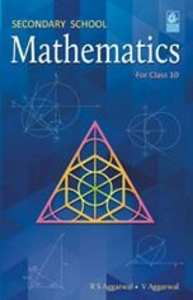## Chapter 4: Triangles

Exercises 1Exercises 2Exercises 3Exercises 4Exercises 5
Exercises 1

### RS Aggarwal solutions for Secondary School Class 10 Maths Chapter 4 Triangles Exercises 1

Exercises 1 | Q 1.1

D and E are points on the sides AB and AC respectively of a ΔABC such that DE║BC.
If AD = 3.6cm, AB = 10cm and AE = 4.5cm, find EC and AC.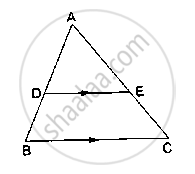Exercises 1 | Q 1.1

D and E are points on the sides AB and AC respectively of a ΔABC such that DE║BC

If AD = 3.6cm, AB = 10cm and AE = 4.5cm, find EC and AC.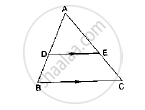Exercises 1 | Q 1.2

D and E are points on the sides AB and AC respectively of a ΔABC such that DE║BC.

If AB = 13.3cm, AC = 11.9cm and EC = 5.1cm, find AD.Exercises 1 | Q 1.3

D and E are points on the sides AB and AC respectively of a ΔABC such that DE║BC.

If (AD)/(DB) = 4/7 and AC = 6.6cm, find AE.Exercises 1 | Q 1.4

D and E are points on the sides AB and AC respectively of a ΔABC such that DE║BC.

If (AD)/(AB) = 8/15 and EC = 3.5cm, find AE.Exercises 1 | Q 2.1

D and E are points on the sides AB and AC respectively of a ΔABC such that DE║BC. Find the value of x, when

AD = x cm, DB = (x – 2) cm, AE = (x + 2) cm and EC = (x – 1) cm.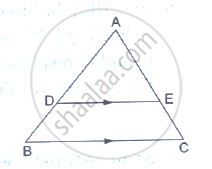Exercises 1 | Q 2.2

D and E are points on the sides AB and AC respectively of a ΔABC such that DE║BC. Find the value of x, when

AD = 4cm, DB = (x – 4) cm, AE = 8cm and EC = (3x – 19) cm.Exercises 1 | Q 2.3

D and E are points on the sides AB and AC respectively of a ΔABC such that DE║BC. Find the value of x, when

AD = (7x – 4) cm, AE = (5x – 2) cm, DB = (3x + 4) cm and EC = 3x cm.

Exercises 1 | Q 3.1

D and E are points on the sides AB and AC respectively of a ΔABC. In each of the following  cases, determine whether DE║BC or not.

AD = 5.7cm, DB = 9.5cm, AE = 4.8cm and EC = 8cm.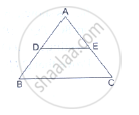Exercises 1 | Q 3.2

D and E are points on the sides AB and AC respectively of a ΔABC. In each of the following cases, determine whether DE║BC or not.

AB = 11.7cm, AC = 11.2cm, BD = 6.5cm and AE = 4.2cm.

Exercises 1 | Q 3.3

D and E are points on the sides AB and AC respectively of a ΔABC. In each of the following cases, determine whether DE║BC or not.

AB = 10.8cm, AD = 6.3cm, AC = 9.6cm and EC = 4cm.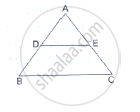Exercises 1 | Q 3.4

D and E are points on the sides AB and AC respectively of a ΔABC. In each of the following cases, determine whether DE║BC or not.

AD = 7.2cm, AE = 6.4cm, AB = 12cm and AC = 10cm.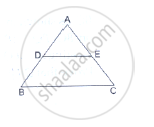Exercises 1 | Q 4.1

In a ΔABC, AD is the bisector of ∠A.

If AB = 6.4cm, AC = 8cm and BD = 5.6cm, find DC.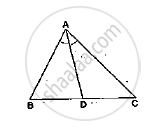Exercises 1 | Q 4.2

In a ΔABC, AD is the bisector of ∠A.

If AB = 10cm, AC = 14cm and BC = 6cm, find BD and DC.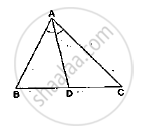Exercises 1 | Q 4.3

In a ΔABC, AD is the bisector of ∠A.

If AB = 5.6cm, BD = 3.2cm and BC = 6cm, find AC.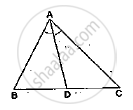Exercises 1 | Q 4.4

In a ΔABC, AD is the bisector of ∠A.

If AB = 5.6cm, AC = 4cm and DC = 3cm, find BC.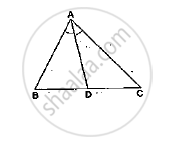Exercises 1 | Q 5

M is a point on the side BC of a parallelogram ABCD. DM when produced meets AB produced at N. Prove that

(1) (DM)/(MN)=(DC)/(BN)

(2) (DN)/(DM)=(AN)/(DC)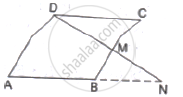Exercises 1 | Q 6

Show that the line segment which joins the midpoints of the oblique sides of a trapezium is parallel sides

Exercises 1 | Q 7

In the given figure, ABCD is a trapezium in which AB║DC and its diagonals intersect at O. If AO = (5x – 7), OC = (2x + 1) , BO = (7x – 5) and OD = (7x + 1), find the value of x.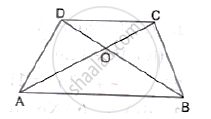Exercises 1 | Q 8

InΔ  ABC , M and N are points on the sides AB and AC respectively such that BM= CN. If ∠B = ∠C then show that MN||BC

Exercises 1 | Q 9

ΔABC and ΔDBC lie on the same side of BC, as shown in the figure. From a point P on BC, PQ||AB and PR||BD are drawn, meeting AC at Q and CD at R respectively. Prove that QR||AD.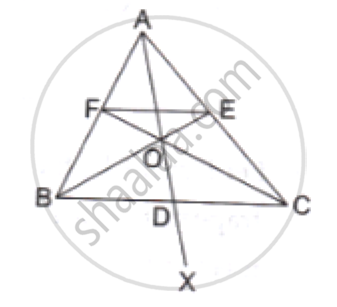Exercises 1 | Q 10

In the given figure, side BC of a ΔABC is bisected at D
and O is any point on AD. BO and CO produced meet
AC and AB at E and F respectively, and AD is
produced to X so that D is the midpoint of OX.
Prove that AO : AX = AF : AB and show that EF║BC.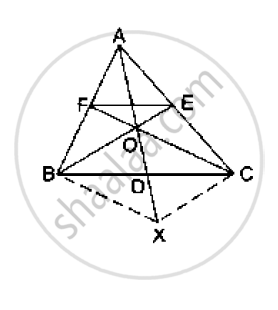Exercises 1 | Q 11

ABCD is a parallelogram in which P is the midpoint of DC and Q is a point on AC such that CQ = 1/4 AC. If PQ produced meets BC at R, prove that R is the midpoint of BC.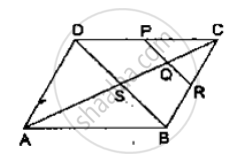Exercises 1 | Q 12

In the adjoining figure, ABC is a triangle in which AB = AC. IF D and E are points on AB and AC respectively such that AD = AE, show that the points B, C, E and D are concyclic.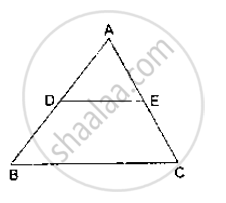Exercises 1 | Q 13

In ΔABC, the bisector of ∠B meets AC at D. A line OQ║AC meets AB, BC and BD at O, Q and R respectively. Show that BP × QR = BQ × PR

Exercises 2

### RS Aggarwal solutions for Secondary School Class 10 Maths Chapter 4 Triangles Exercises 2

Exercises 2 | Q 1.1

In each of the given pairs of triangles, find which pair of triangles are similar. State the similarity criterion and write the similarity relation in symbolic form: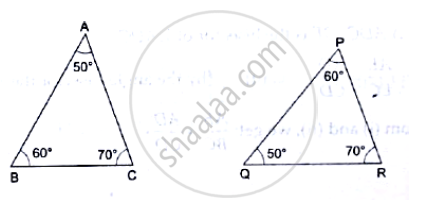Exercises 2 | Q 1.2

In each of the given pairs of triangles, find which pair of triangles are similar. State the similarity criterion and write the similarity relation in symbolic form: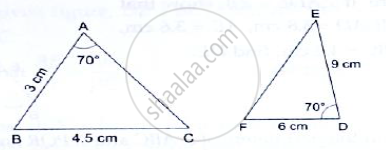Exercises 2 | Q 1.3

In each of the given pairs of triangles, find which pair of triangles are similar. State the similarity criterion and write the similarity relation in symbolic form: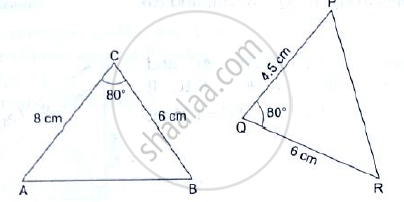Exercises 2 | Q 1.4

In each of the given pairs of triangles, find which pair of triangles are similar. State the similarity criterion and write the similarity relation in symbolic form: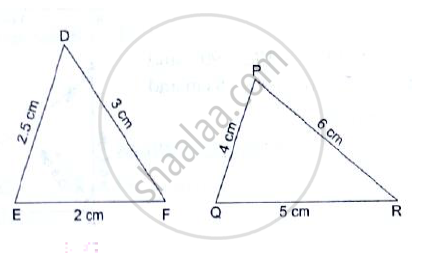Exercises 2 | Q 1.5

In each of the given pairs of triangles, find which pair of triangles are similar. State the similarity criterion and write the similarity relation in symbolic form: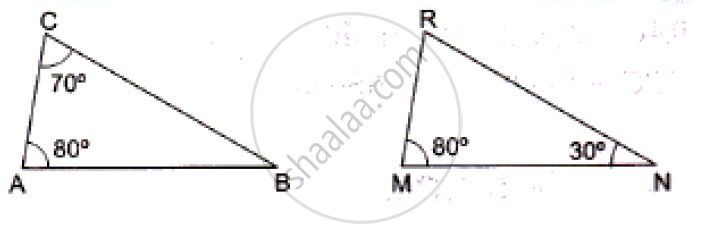Exercises 2 | Q 2

In the given figure, ΔODC~ΔOBA, ∠BOC = 115° and ∠CDO = 700.
Find (i) ∠DCO (ii) ∠DCO (iii) ∠OAB (iv) ∠OBA.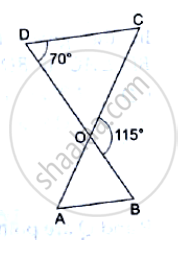Exercises 2 | Q 3

In the given figure, ΔOAB ~ ΔOCD. If AB = 8cm, BO = 6.4cm, OC = 3.5cm and CD = 5cm, find (i) OA (ii) DO.

Exercises 2 | Q 4

In the given figure, if ∠ADE = ∠B, show that ΔADE ~ ΔABC. If AD = 3.8cm, AE = 3.6cm, BE = 2.1cm and BC = 4.2cm, find DE.

Exercises 2 | Q 5

The perimeter of two similar triangles ABC and PQR are 32cm and 24cm respectively. If PQ = 12cm, find AB.

Exercises 2 | Q 6

The corresponding sides of two similar triangles ABC and DEF are BC = 9.1cm and EF = 6.5cm. If the perimeter of ΔDEF is 25cm, find the perimeter of ΔABC.

Exercises 2 | Q 7

In the given figure, ∠CAB = 90° and AD⊥BC. Show that ΔBDA ~ ΔBAC. If AC = 75cm, AB = 1m and BC = 1.25m, find AD.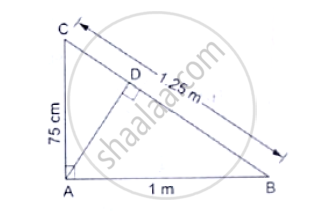Exercises 2 | Q 8

In the given figure, ∠ABC = 90° and BD⊥AC. If AB = 5.7cm, BD = 3.8cm and CD = 5.4cm, find BC.

Exercises 2 | Q 9

In the given figure, ∠ABC = 90° and BD⊥AC. If BD = 8cm, AD = 4cm, find CD.

Exercises 2 | Q 10

P and Q are points on the sides AB and AC respectively of a ΔABC. If AP = 2cm, PB = 4cm, AQ = 3cm and QC = 6cm, show that BC = 3PQ.

Exercises 2 | Q 11

ABCD is parallelogram and E is a point on BC.  If the diagonal BD intersects AE at F, prove that AF × FB = EF × FD.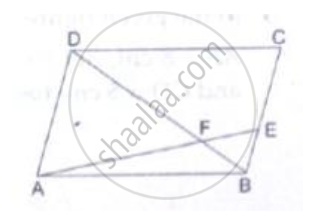Exercises 2 | Q 12

In the given figure, DB⊥BC, DE⊥AB and AC⊥BC.
Prove that  (BE)/(DE)=(AC)/(BC)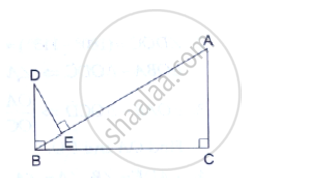Exercises 2 | Q 13

A vertical pole of length 7.5cm casts a shadow 5m long on the ground and at the same time a tower casts a shadow 24m long. Find the height of the tower.

Exercises 2 | Q 14

In an isosceles ΔABC, the base AB is produced both ways in P and Q such that
AP × BQ = AC2.
Prove that ΔACP~ΔBCQ.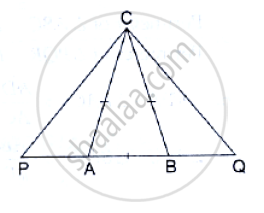Exercises 2 | Q 15

In the given figure, ∠1 = ∠2 and (AC)/(BD)=(CB)/(CE)   Prove that Δ ACB ~ Δ DCE.

Exercises 2 | Q 16

ABCD is a quadrilateral in which AD = BC. If P, Q, R, S be the midpoints of AB, AC, CD and BD respectively, show that PQRS is a rhombus.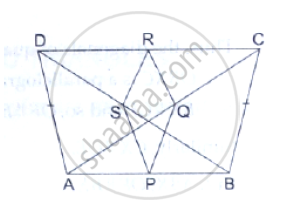Exercises 2 | Q 17

In a circle, two chords AB and CD intersect at a point P inside the circle. Prove that
(a) ΔPAC ∼PDB (b) PA. PB= PC.PD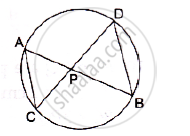Exercises 2 | Q 18

Two chords AB and CD of a circle intersect at a point P outside the circle.
Prove that: (i) Δ PAC ~ Δ PDB  (ii) PA. PB = PC.PD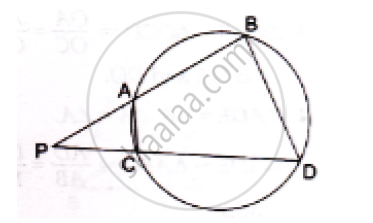Exercises 2 | Q 19

In a right triangle ABC, right angled at B, D is a point on hypotenuse such that BD ⊥ AC , if DP ⊥ AB and DQ ⊥ BC then prove that
(a)  DQ^2  Dp.QC (b)  DP ^2 DQ.AP 2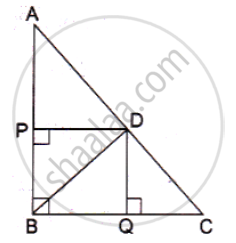Exercises 3

### RS Aggarwal solutions for Secondary School Class 10 Maths Chapter 4 Triangles Exercises 3

Exercises 3 | Q 1

ΔABC~ΔDEF and their areas are respectively 64 cm2 and 121cm2. If EF = 15.4cm, find BC.

Exercises 3 | Q 2

The areas of two similar triangles ABC and PQR are in the ratio 9:16. If BC = 4.5cm, find the length of QR.

Exercises 3 | Q 3

ΔABC~ΔPQR and ar(ΔABC) = 4, ar(ΔPQR) . If BC = 12cm, find QR.

Exercises 3 | Q 4

The areas of two similar triangles are 169cm2 and 121cm2 respectively. If the longest side of the larger triangle is 26cm, find the longest side of the smaller triangle.

Exercises 3 | Q 5

ΔABC ~ ΔDEF and their areas are respectively 100cm^2 and 49cm2. If the altitude of ΔABC is 5cm, find the corresponding altitude of ΔDEF.

Exercises 3 | Q 6

The corresponding altitudes of two similar triangles are 6cm and 9cm respectively. Find the ratio of their areas.

Exercises 3 | Q 7

The areas of two similar triangles are 81cm^2 and 49cm^2 respectively. If the altitude of the first triangle is 6.3cm, find the corresponding altitude of the other.

Exercises 3 | Q 8

The areas of two similar triangles are 64cm^2 and 100cm^2 respectively. If a median of the smaller triangle is 5.6cm, find the corresponding median of the other.

Exercises 3 | Q 9

In the given figure, ABC is a triangle and PQ is a straight line meeting AB in P and AC in Q. If AP = 1cm, PB = 3cm, AQ = 1.5cm, QC = 4.5cm, prove that area of ΔAPQ is 116 of the area of ΔABC.

Exercises 3 | Q 10

In the given figure, DE║BC. If DE = 3cm, BC = 6cm and ar(ΔADE) = 15cm^2, find the area of ΔABC.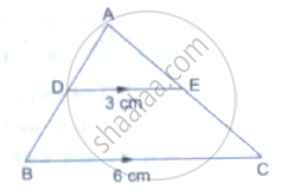Exercises 3 | Q 11

ΔABC is right angled at A and AD⊥BC. If BC = 13cm and AC = 5cm, find the ratio of the areas of ΔABC and ΔADC.

Exercises 3 | Q 12

In the given figure, DE║BC and DE: BC = 3:5. Calculate the ratio of the areas of ΔADE and the trapezium BCED.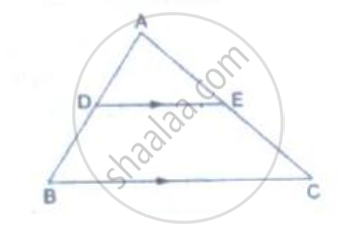Exercises 3 | Q 13

In ΔABC, D and E are the midpoints of AB and AC respectively. Find the ratio of the areas of ΔADE and ΔABC.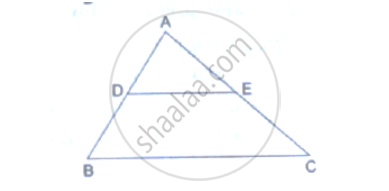Exercises 4

### RS Aggarwal solutions for Secondary School Class 10 Maths Chapter 4 Triangles Exercises 4

Exercises 4 | Q 1.1

The sides of certain triangles are given below. Determine which of them right triangles are.

9cm, 16cm, 18cm

Exercises 4 | Q 1.2

The sides of certain triangles are given below. Determine which of them right triangles are.

7cm, 24cm, 25cm

Exercises 4 | Q 1.3

The sides of certain triangles are given below. Determine which of them right triangles are.

1.4cm, 4.8cm, 5cm

Exercises 4 | Q 1.4

The sides of certain triangles are given below. Determine which of them right triangles are.

1.6cm, 3.8cm, 4cm

Exercises 4 | Q 1.5

The sides of certain triangles are given below. Determine which of them right triangles are.

(a – 1) cm, 2sqrta cm, (a + 1) cm

Exercises 4 | Q 2

A man goes 80m due east and then 150m due north. How far is he from the starting point?

Exercises 4 | Q 3

A man goes 10m due south and then 24m due west. How far is he from the starting point?

Exercises 4 | Q 4

A 13m long ladder reaches a window of a building 12m above the ground. Determine the distance of the foot of the ladder from the building.

Exercises 4 | Q 5

A ladder is placed in such a way that its foot is at a distance of 15m from a wall and its top reaches a window 20m above the ground. Find the length of the ladder.

Exercises 4 | Q 6

Prove that the diagonals of a rectangle ABCD, with vertices A(2, -1), B(5, -1), C(5, 6) and D(2, 6), are equal and bisect each other.

Exercises 4 | Q 6

Two vertical poles of height 9m and 14m stand on a plane ground. If the distance between their feet is 12m, find the distance between their tops.

Exercises 4 | Q 7

A guy wire attached to a vertical pole of height 18 m is 24m long and has a stake attached to the other end. How far from the base of the pole should the stake be driven so that the wire will be taut?

Exercises 4 | Q 8

In the given figure, O is a point inside a ΔPQR such that ∠PQR such that ∠POR = 90°, OP = 6cm and OR = 8cm. If PQ = 24cm and QR = 26cm, prove that ΔPQR is right-angled.

Exercises 4 | Q 9

ΔABC is an isosceles triangle with AB = AC = 13cm. The length of altitude from A on BC is 5cm. Find BC.

Exercises 4 | Q 10

Find the length of altitude AD of an isosceles ΔABC in which AB = AC = 2a units and BC = a units.

Exercises 4 | Q 11

ΔABC is am equilateral triangle of side 2a units. Find each of its altitudes.

Exercises 4 | Q 12

Find the height of an equilateral triangle of side 12cm.

Exercises 4 | Q 13

Find the length of a diagonal of a rectangle whose adjacent sides are 30cm and 16cm.

Exercises 4 | Q 14

Find the length of each side of a rhombus whose diagonals are 24cm and 10cm long.

Exercises 4 | Q 15

In ΔABC, D is the midpoint of BC and AE⊥BC. If AC>AB, show that AB^2= AD^2+1/4 BC^2 −BC.DE

Exercises 4 | Q 16

In the given figure, ∠ACB  90° CD ⊥ AB Prove that (BC^2)/(AC^2)=(BD)/(AD)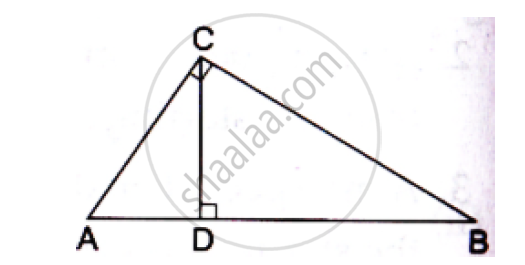Exercises 4 | Q 17

In the given figure, D is the midpoint of side BC and AE⊥BC. If BC = a, AC = b, AB = c, AD = p and AE = h, prove that

(i)B^2=p^2+ax+a^2/x
(ii) c^2=p^2-ax+a^2/x
(iii) b^2+c^2=2p^2+a^2/2

(iv)b^2-c^2=2ax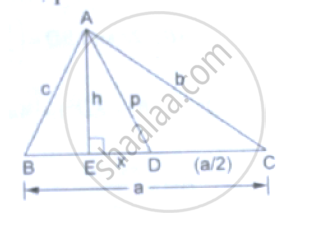Exercises 4 | Q 18

In ΔABC, AB = AC. Side BC is produced to D. Prove that AD^2−AC^2= BD.CD

Exercises 4 | Q 19

ABC is an isosceles triangle, right-angled at B. Similar triangles ACD and ABE are constructed on sides AC and AB. Find the ratio between the areas of ΔABE and ΔACD.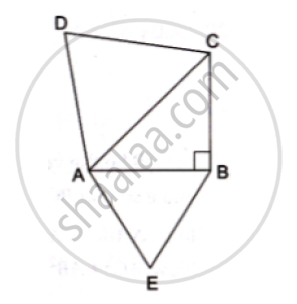Exercises 4 | Q 20

An aeroplane leaves an airport and flies due north at a speed of 1000km per hour. At the same time, another aeroplane leaves the same airport and flies due west at a speed of 1200 km per hour. How far apart will be the two planes after 1 1/2  hours?

Exercises 4 | Q 21

In a ABC , AD is a median and AL ⊥ BC .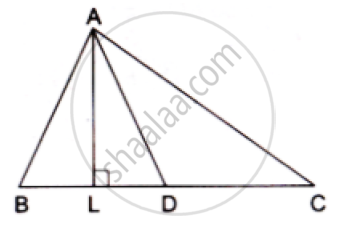Prove that

(a) AC^2=AD^2+BC  DL+((BC)/2)^2

(b) AB^2=AD^2-BC  DL+((BC)/2)^2

(c) AC^2+AB^2=2.AD^2+1/2BC^2

Exercises 5

### RS Aggarwal solutions for Secondary School Class 10 Maths Chapter 4 Triangles Exercises 5

Exercises 5 | Q 1

State the two properties which are necessary for given two triangles to be similar.

Exercises 5 | Q 2

State the basic proportionality theorem.

Exercises 5 | Q 3

State and converse of Thale’s theorem.

Exercises 5 | Q 4

State the midpoint theorem

Exercises 5 | Q 5

State the AAA-similarity criterion

Exercises 5 | Q 6

State the AA-similarity criterion

Exercises 5 | Q 7

State the SSS-similarity criterion for similarity of triangles

Exercises 5 | Q 8

State the SAS-similarity criterion

Exercises 5 | Q 9

State Pythagoras theorem

Exercises 5 | Q 10

State the converse of Pythagoras theorem.

Exercises 5 | Q 11

If D, E, F are the respectively the midpoints of sides BC, CA and AB of ΔABC. Find the ratio of the areas of ΔDEF and ΔABC.

Exercises 5 | Q 12

Two triangles ABC and PQR are such that AB = 3 cm, AC = 6cm, ∠𝐴 = 70°, PR = 9cm ∠𝑃 = 70° and PQ = 4.5 cm. Show that ΔABC ∼ΔPQR and state that similarity criterion.

Exercises 5 | Q 13

In ΔABC~ΔDEF such that 2AB = DE and BC = 6cm, find EF.

Exercises 5 | Q 14

In the given figure, DE║BC such that AD = x cm, DB = (3x + 4) cm, AE = (x + 3) cm and EC = (3x + 19) cm. Find the value of x.

Exercises 5 | Q 15

A ladder 10m long reaches the window of a house 8m above the ground. Find the distance of the foot of the ladder from the base of the wall.

Exercises 5 | Q 16

Find the length of the altitude of an equilateral triangle of side 2a cm.

Exercises 5 | Q 17

ΔABC~ΔDEF such that ar(ΔABC) = 64 cm2 and ar(ΔDEF) = 169cm^2. If BC = 4cm, find EF.

Exercises 5 | Q 18

In a trapezium ABCD, it is given that AB║CD and AB = 2CD. Its diagonals AC and BD intersect at the point O such that ar(ΔAOB) = 84cm2. Find ar(ΔCOD).

Exercises 5 | Q 19

The corresponding sides of two similar triangles are in the ratio 2: 3. If the area of the smaller triangle is 48cm^2, find the area of the larger triangle.

Exercises 5 | Q 20

In an equilateral triangle with side a, prove that area = sqrt3/4 𝑎2

Exercises 5 | Q 21

Find the length of each side of a rhombus whose diagonals are 24cm and 10cm long.

Exercises 5 | Q 22

Two triangles DEF an GHK are such that ∠D = 48° and ∠H = 57° . If ΔDEF ∼GHK then find the measures of ∠F

Exercises 5 | Q 23

In the given figure MN|| BC and AM: MB= 1: 2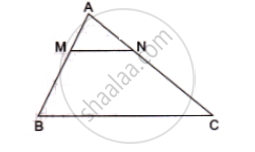find     (area(ΔAMN))/(area(ΔABC))

Exercises 5 | Q 24

In triangle BMP and CNR it is given that PB= 5 cm, MP = 6cm BM = 9 cm and NR = 9cm. If ΔBMP∼ ΔCNR then find the perimeter of ΔCNR

Exercises 5 | Q 25

Each of the equal sides of an isosceles triangle is 25 cm. Find the length of its altitude if the  base is 14 cm.

Exercises 5 | Q 26

A man goes 12m due south and then 35m due west. How far is he from the starting point.

Exercises 5 | Q 27

If the lengths of the sides BC, CA and AB of a ΔABC are a, b and c respectively and AD is the bisector ∠A then find the lengths of BD and DC

Exercises 5 | Q 28

In the given figure, ∠AMN = ∠MBC = 76° . If p, q and r are the lengths of AM, MB and BC respectively then express the length of MN of terms of P, q and r.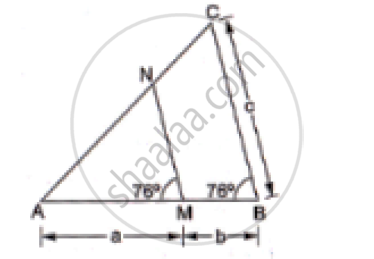Exercises 5 | Q 29

Find the length of each side of a rhombus are 40 cm and 42 cm. find the length of each side of the rhombus.

Exercises 5 | Q 30.01

For each of the following statements state whether true(T) or false (F)

Two circles with different radii are similar.

Exercises 5 | Q 30.02

For each of the following statements state whether true(T) or false (F)

any two rectangles are similar

Exercises 5 | Q 30.03

For each of the following statements state whether true(T) or false (F)

if two triangles are similar then their corresponding angles are equal and their corresponding sides are equal

Exercises 5 | Q 30.04

For each of the following statements state whether true(T) or false (F)

The length of the line segment joining the midpoints of any two sides of a triangles is equal to half the length of the third side.

Exercises 5 | Q 30.05

For each of the following statements state whether true(T) or false (F)

In a ΔABC , AB = 6 cm, ∠A = 45° and AC = 8 cm and in a ΔDEF , DF = 9 cm ∠D = 45°  and DE= 12 cm then ΔABC ∼ΔDEF

Exercises 5 | Q 30.06

For each of the following statements state whether true(T) or false (F)

the polygon formed by joining the midpoints of the sides of a quadrilateral is a rhombus.

Exercises 5 | Q 30.07

For each of the following statements state whether true(T) or false (F)

the ratio of the perimeter of two similar triangles is the same as the ratio of the their corresponding medians.

Exercises 5 | Q 30.09

If O is Any Point Inside a Rectangle Abcd Then Oa^2+Oc^2=Ob^2+Od^2

Exercises 5 | Q 30.1

For each of the following statements state whether true(T) or false (F)

The sum of the squares on the sides of a rhombus is equal to the sum of the squares on its diagonals.

## Chapter 4: Triangles

Exercises 1Exercises 2Exercises 3Exercises 4Exercises 5## RS Aggarwal solutions for Secondary School Class 10 Maths chapter 4 - Triangles

RS Aggarwal solutions for Secondary School Class 10 Maths chapter 4 (Triangles) include all questions with solution and detail explanation. This will clear students doubts about any question and improve application skills while preparing for board exams. The detailed, step-by-step solutions will help you understand the concepts better and clear your confusions, if any. Shaalaa.com has the CBSE Secondary School Class 10 Maths solutions in a manner that help students grasp basic concepts better and faster.

Further, we at Shaalaa.com provide such solutions so that students can prepare for written exams. RS Aggarwal textbook solutions can be a core help for self-study and acts as a perfect self-help guidance for students.

Concepts covered in Secondary School Class 10 Maths chapter 4 Triangles are Similar Figures, Similarity of Triangles, Basic Proportionality Theorem (Thales Theorem), Criteria for Similarity of Triangles, Areas of Similar Triangles, Application of Pythagoras Theorem in Acute Angle and Obtuse Angle, Triangles Examples and Solutions, Angle Bisector, Ratio of Sides of Triangle, Right-angled Triangles and Pythagoras Property, Similarity of Triangles, Similarity of Triangles, Similar Figures, Similarity of Triangles, Basic Proportionality Theorem (Thales Theorem), Criteria for Similarity of Triangles, Areas of Similar Triangles, Application of Pythagoras Theorem in Acute Angle and Obtuse Angle, Triangles Examples and Solutions, Angle Bisector, Ratio of Sides of Triangle, Right-angled Triangles and Pythagoras Property, Similarity of Triangles, Similarity of Triangles.

Using RS Aggarwal Class 10 solutions Triangles exercise by students are an easy way to prepare for the exams, as they involve solutions arranged chapter-wise also page wise. The questions involved in RS Aggarwal Solutions are important questions that can be asked in the final exam. Maximum students of CBSE Class 10 prefer RS Aggarwal Textbook Solutions to score more in exam.

Get the free view of chapter 4 Triangles Class 10 extra questions for Secondary School Class 10 Maths and can use Shaalaa.com to keep it handy for your exam preparation